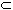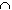#Interactive Real Analysis - part of MathCS.org

Next | Previous | Glossary | Map | Discussion

## Proposition 5.2.11: Perfect sets are Uncountable

Every non-empty perfect set must be uncountable.

### Proof:

If S is perfect, it consists of accumulation points, and therefore can not be finite. Therefore it is either countable or uncountable. Suppose S was countable and could be written as
• S = { x1, x2, x3, ...}
The interval U1 = (x1 - 1, x1 + 1) is a neighborhood of x1. Since x1 must be an accumulation point of S, there are infinitely many elements of S contained in U1.

Take one of those elements, say x2 and take a neighborhood U2 of x2 such that closure( U2 ) is contained in U1 and x1 is not contained in closure( U2 ). Again, x2 is an accumulation point of S, so that the neighborhood U2 contains infinitely many elements of S.

Select an element, say x3, and take a neighborhood U3 of x3 such that closure( U3 ) is contained in U2 but x1 and x2 are not contained in closure( U3 ) .

Continue in that fashion: we can find sets Un and points xn such that:

• closure( Un+1 )Un
• xj is not contained in Un for all 0 < j < n
• xn is contained in Un
Now consider the set
• V =( closure( Un )S )
Then each set closure( Un )S ) is closed and bounded, hence compact. Also, by construction, ( closure( Un+1 )S )(closure( Un)S ). Therefore, by the above result, V is not empty. But which element of S should be contained in V ? It can not be x1, because x1 is not contained in closure( U2 ). It can not be x2 because x2 is not in closure( U3 ), and so forth.

Hence, none of the elements { x1, x2, x3, ... } can be contained in V. But V is non-empty, so that it must contain an element not in this list. That means, however, that S is not countable.Next | Previous | Glossary | Map | Discussion